10 Motion of a charged particle in the magnetic field
Оценка 4.9

# 10 Motion of a charged particle in the magnetic field

Оценка 4.9
pptx
07.05.2020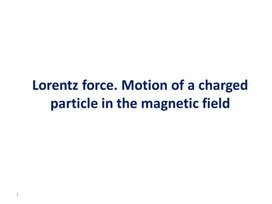10 Motion of a charged particle in the magnetic field.pptx

## Lorentz force. Motion of a charged particle in the magnetic field 1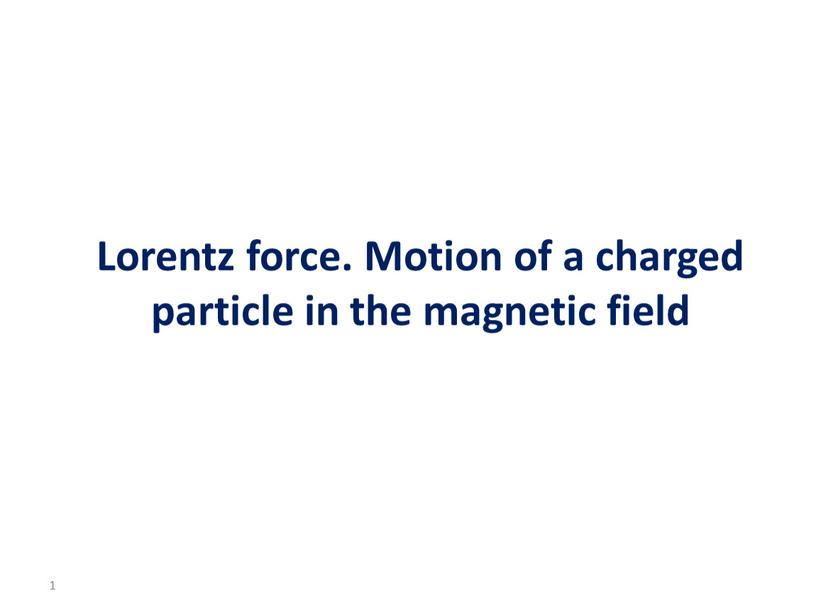Lorentz force. Motion of a charged particle in the magnetic field

1

## Learning objective To investigate the effect of a magnetic field on moving charged particles; 2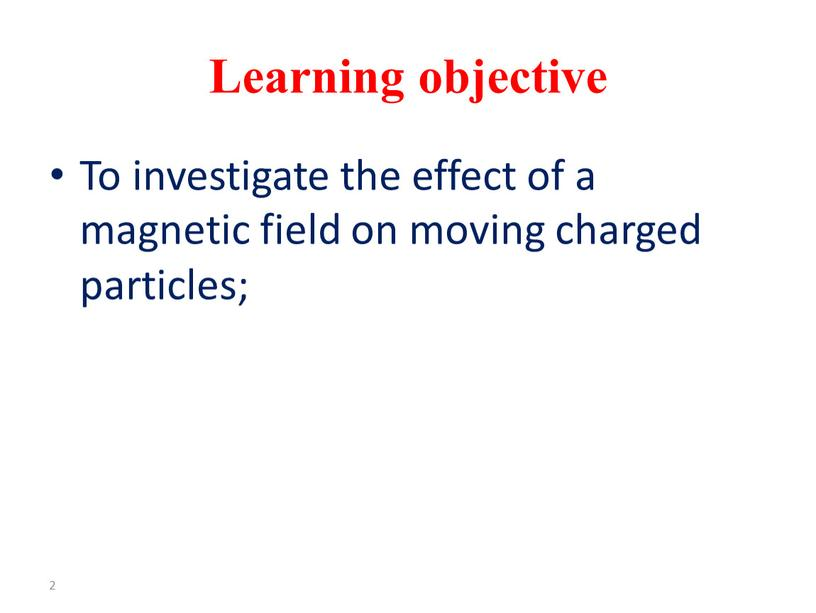Learning objective

To investigate the effect of a magnetic field on moving charged particles;

2

## Motivation If the speed of the particle and radius of its path are known, then the specific charge/the ratio of charge to mass can be…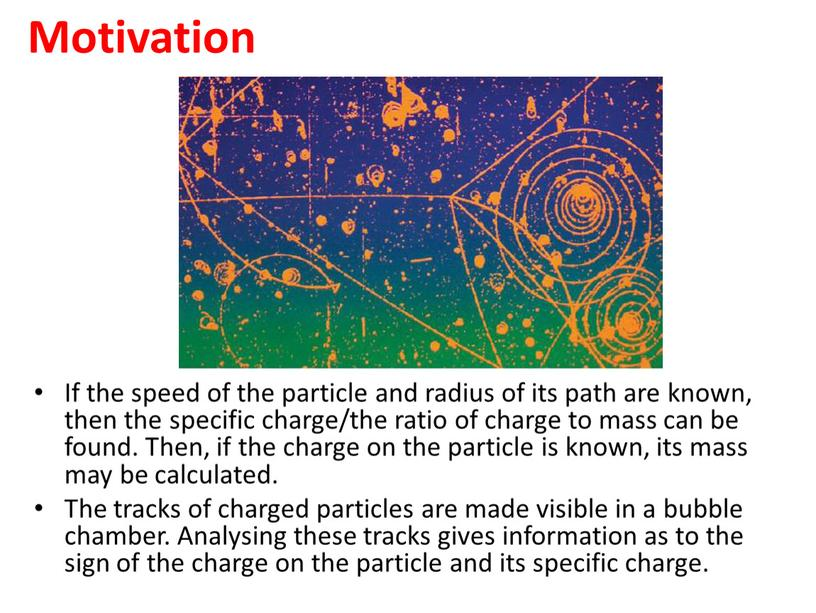Motivation

If the speed of the particle and radius of its path are known, then the specific charge/the ratio of charge to mass can be found. Then, if the charge on the particle is known, its mass may be calculated.
The tracks of charged particles are made visible in a bubble chamber. Analysing these tracks gives information as to the sign of the charge on the particle and its specific charge.

Thompson’s experiment

In 1897 JJ Thomson set out to prove that cathode rays originating from a heated cathode (electron gun), were actually a stream of small negatively charged particles called electrons.
Electrons are accelerated from the cathode.
They are deflected by electric and magnetic fields.
The beam of electrons strikes a fluorescent screen.
e/m was measured.

## G) Group work GROUP 1: The charge-to-mass ratio of an electron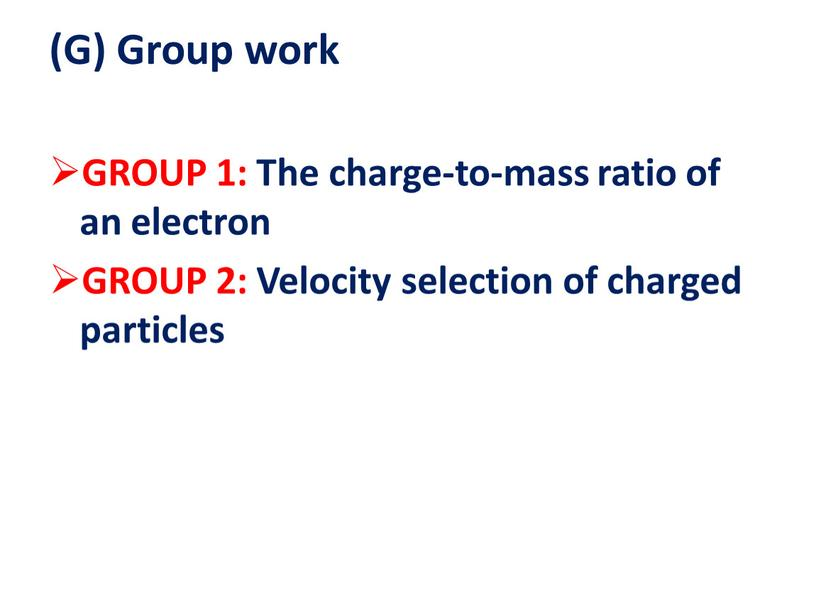(G) Group work

GROUP 1: The charge-to-mass ratio of an electron
GROUP 2: Velocity selection of charged particles

The charge-to-mass ratio of an electron

Since the electrons are moving in a circle, there must be centripetal force
The magnetic force is acting as the centripetal force: FM = FC
Therefore,  Bqv = mv2/r
Re-arranging the terms,
q/m = v/Br

## The ratio e/m for an electron – its specific charge – may be determined using a fine-beam tube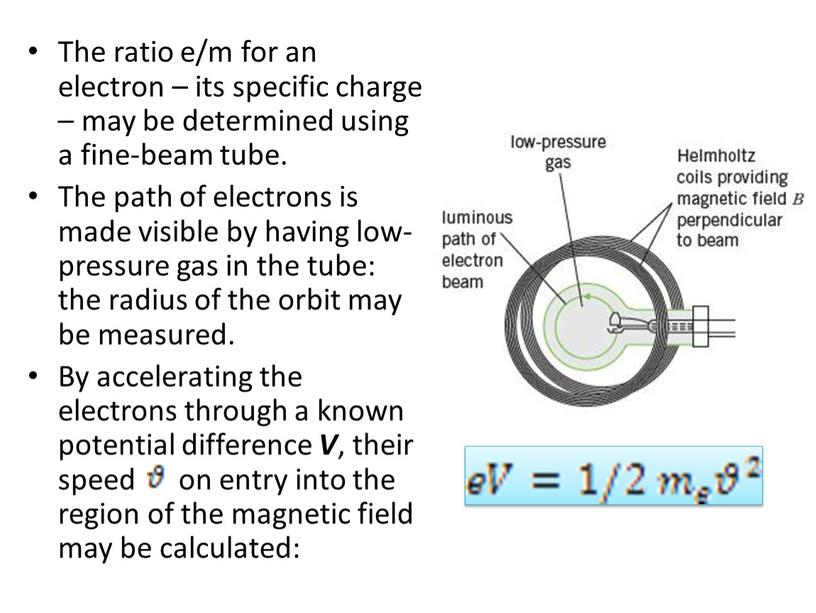The ratio e/m for an electron – its specific charge – may be determined using a fine-beam tube.
The path of electrons is made visible by having low-pressure gas in the tube: the radius of the orbit may be measured.
By accelerating the electrons through a known potential difference V, their speed on entry into the region of the magnetic field may be calculated:

## The magnetic field is provided by a pair of current-carrying coils (Helmholtz coils,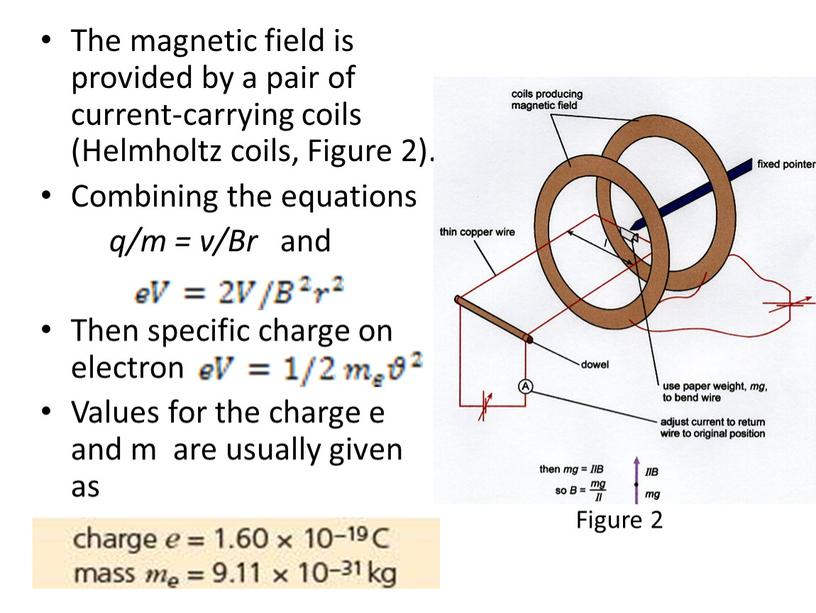The magnetic field is provided by a pair of current-carrying coils (Helmholtz coils, Figure 2).
Combining the equations
q/m = v/Br and

Then specific charge on electron
Values for the charge e and m are usually given as

Figure 2

## Velocity Selector Used when all the particles need to move with the same velocity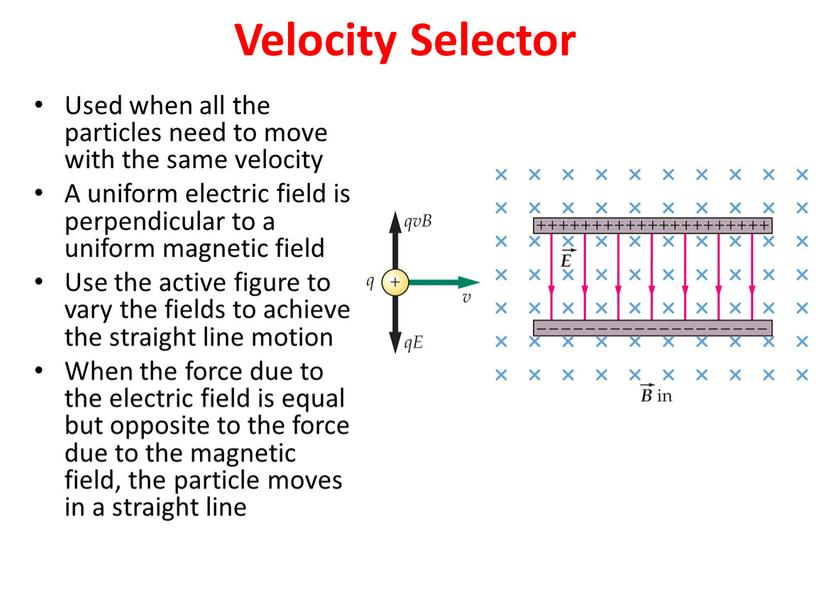Velocity Selector

Used when all the particles need to move with the same velocity
A uniform electric field is perpendicular to a uniform magnetic field
Use the active figure to vary the fields to achieve the straight line motion
When the force due to the electric field is equal but opposite to the force due to the magnetic field, the particle moves in a straight line

## Net force: The forces balance if the speed of the particle is related to the field strengths by qvB = qE v =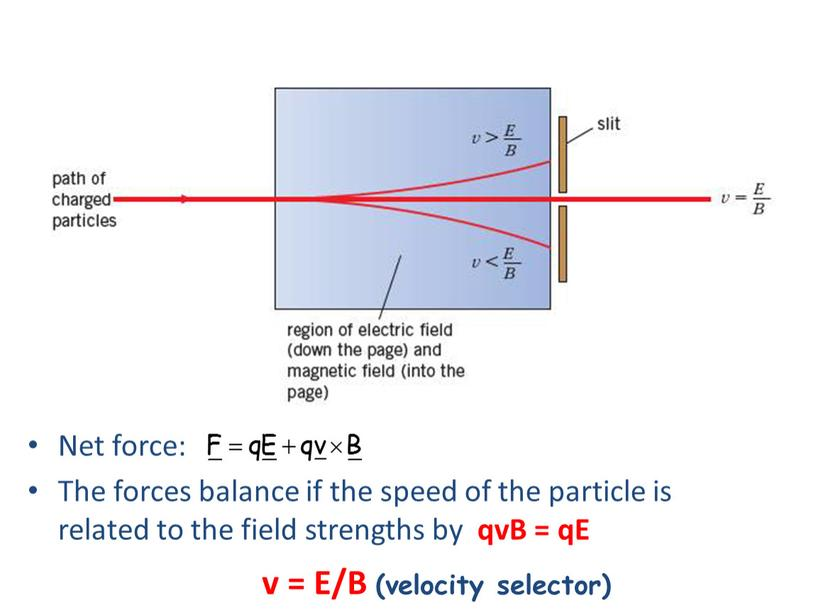Net force:
The forces balance if the speed of the particle is related to the field strengths by qvB = qE

v = E/B (velocity selector)

## Mass Spectrometer A mass spectrometer separates ions according to their mass-to-charge ratio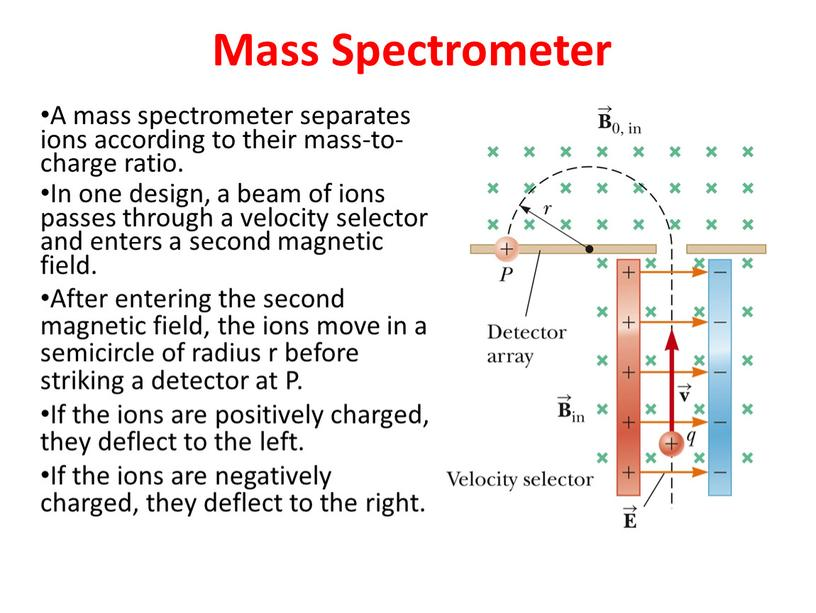Mass Spectrometer

A mass spectrometer separates ions according to their mass-to-charge ratio.
In one design, a beam of ions passes through a velocity selector and enters a second magnetic field.
After entering the second magnetic field, the ions move in a semicircle of radius r before striking a detector at P.
If the ions are positively charged, they deflect to the left.
If the ions are negatively charged, they deflect to the right.

## The mass to charge (m/q) ratio can be determined by measuring the radius of curvature and knowing the magnetic and electric field magnitudes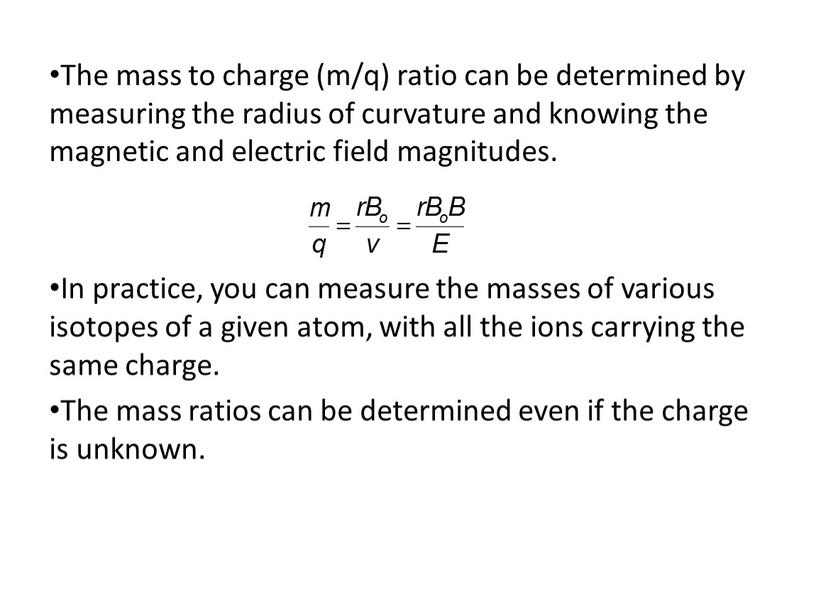The mass to charge (m/q) ratio can be determined by measuring the radius of curvature and knowing the magnetic and electric field magnitudes.

In practice, you can measure the masses of various isotopes of a given atom, with all the ions carrying the same charge.
The mass ratios can be determined even if the charge is unknown.

## This question is about the velocity selector shown in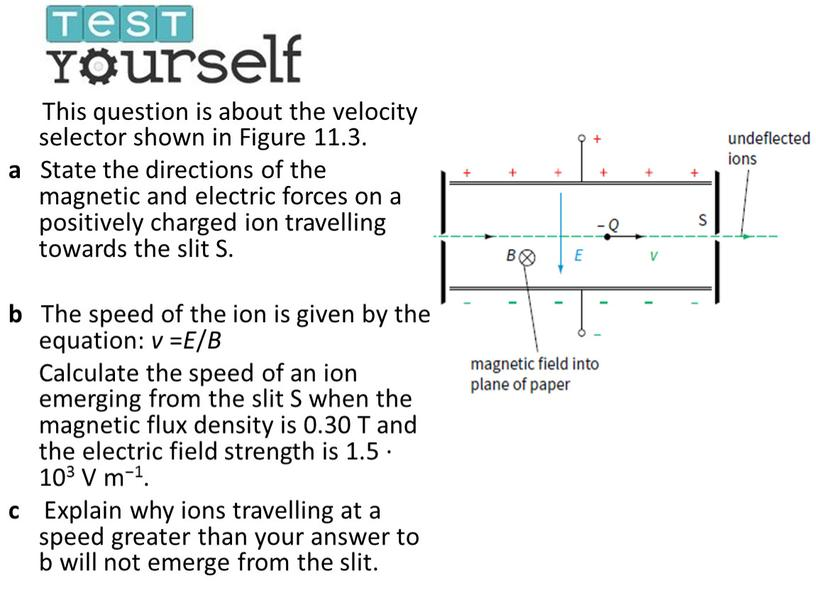This question is about the velocity selector shown in Figure 11.3.
a State the directions of the magnetic and electric forces on a positively charged ion travelling towards the slit S.

b The speed of the ion is given by the equation: v =E/B
Calculate the speed of an ion emerging from the slit S when the magnetic flux density is 0.30 T and the electric field strength is 1.5 ∙ 103 V m−1.
c Explain why ions travelling at a speed greater than your answer to b will not emerge from the slit.

## Answer Magnetic force - upwards and electric force – downwards; v =1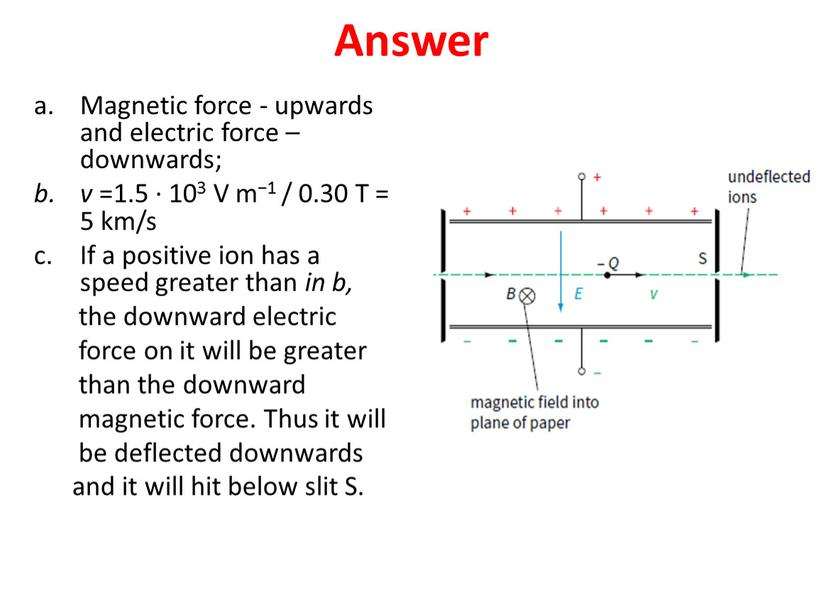Magnetic force - upwards and electric force – downwards;
v =1.5 ∙ 103 V m−1 / 0.30 T = 5 km/s
If a positive ion has a speed greater than in b,
the downward electric
force on it will be greater
than the downward
magnetic force. Thus it will
be deflected downwards
and it will hit below slit S.

Reflection

What has been learned
What remained unclear
What is necessary to work on

Скачать файл